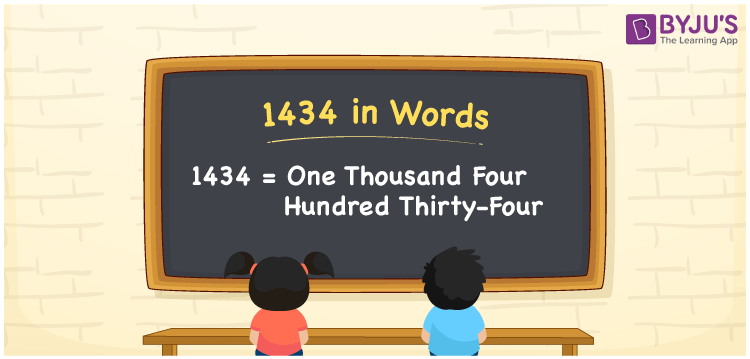# 1434 in Words

1434 in words is written as One Thousand Four Hundred Thirty-Four. For example, Siya purchased an idli maker worth Rs.1434, then you can say, “Siya purchased an idli maker worth Rupees One Thousand Four Hundred Thirty-Four”. 1434 is a cardinal number since it denotes the exact value of something. Place value chart helps us to write number names easily and quickly. Let’s learn the conversion of the number 1434 into words in this article.

 1434 in Words One Thousand Four Hundred Thirty-Four One Thousand Four Hundred Thirty-Four in numerical form 1434

## 1434 in English Words

We generally, express the numbers in words with the help of the English alphabet. Therefore, the number 1434 in English is expressed as One Thousand Four Hundred Thirty-Four.## How to Write 1434 in Words?

1434 is a four-digit number. Let us create a table of four columns to show the place value chart for 1434. The below table depicts the place value chart for 1434.

 Thousands Hundreds Tens Ones 1 4 3 4

Hence, we can write the expanded form as:

1 x Thousand + 4 x Hundred + 3 x Ten + 4 x One

= 1 x 1000 + 4 x 100 + 3 x 10 + 4 x 1

= 1000 + 400 + 30 + 4

= 1434

= One Thousand Four Hundred Thirty-Four

Hence, 1434 in words is written as One Thousand Four Hundred Thirty-Four

Interesting way of writing 1434 in words

1 = One

14 = Fourteen

143 = One Hundred and Forty-Three

1434 = One Thousand Four Hundred Thirty-Four

Thus, the word form of the number 1434 is One Thousand Four Hundred Thirty-Four

1434 is a natural number that precedes 1435 and succeeds 1433

• 1434 in words – One Thousand Four Hundred Thirty-Four
• Is 1434 an odd number? – No
• Is 1434 an even number? – Yes
• Is 1434 a perfect square number? – No
• Is 1434 a perfect cube number? – No
• Is 1434 a prime number? – No
• Is 1434 a composite number? – Yes

## Frequently Asked Questions on 1434 in Words

Q1

### Write 1434 in words.

1434 in words is written as One Thousand Four Hundred Thirty-Four.
Q2

### Simplify 1000 + 434, and express in words.

Simplifying 1000 + 434, we get 1434. Hence, the number 1434 in words is One Thousand Four Hundred Thirty-Four.
Q3

### Write One Thousand Four Hundred Thirty-Four in numbers.

One Thousand Four Hundred Thirty-Four in numbers is 1434.